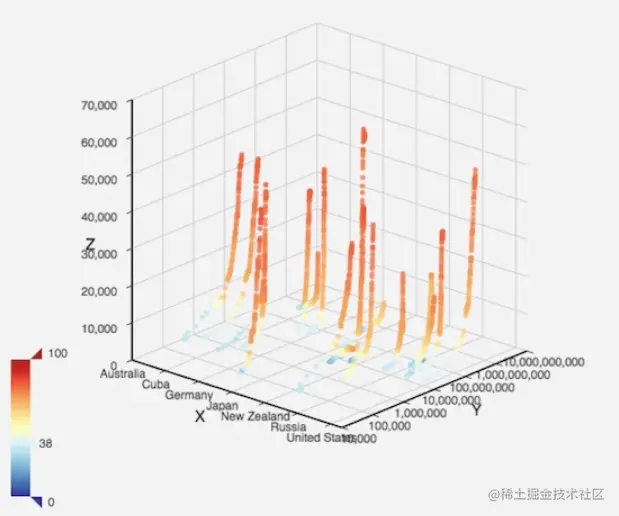﻿ ECharts的三维可视化及在微信小程序中使用示例_JavaScript_脚本之家
JavaScript# ECharts的三维可视化及在微信小程序中使用示例

## 在微信小程序中使用 ECharts

pages目录下的每个文件夹都是一个页面。可以根据情况删除不必要的页面，然后单击应用程序删除json中的相应页面。 如果只复制ec画布目录，则可以参考pages/bar目录中多个文件的写入方法。现在，让我们详细解释一下。

```{
"usingComponents": {
"ec-canvas": "../../ec-canvas/ec-canvas"
}
}
```

```<view class="container">
<ec-canvas id="mychart-dom-bar" canvas-id="mychart-bar" ec="{{ ec }}"></ec-canvas>
</view>
```

```function initChart(canvas, width, height) {
const chart = echarts.init(canvas, null, {
width: width,
height: height
});
canvas.setChart(chart);
var option = {
...
};
chart.setOption(option);
return chart;
}
Page({
data: {
ec: {
onInit: initChart
}
}
});
```

## 三维可视化

```<script src="lib/echarts.min.js"></script>
<script src="lib/echarts-gl.min.js"></script>
```

```var option = {
grid3D: {},
xAxis3D: {},
yAxis3D: {},
zAxis3D: {}
}
``````function makeGaussian(amplitude, x0, y0, sigmaX, sigmaY) {
return function (amplitude, x0, y0, sigmaX, sigmaY, x, y) {
var exponent = -(
( Math.pow(x - x0, 2) / (2 * Math.pow(sigmaX, 2)))
+ ( Math.pow(y - y0, 2) / (2 * Math.pow(sigmaY, 2)))
);
return amplitude * Math.pow(Math.E, exponent);
}.bind(null, amplitude, x0, y0, sigmaX, sigmaY);
}
// 创建一个高斯分布函数
var gaussian = makeGaussian(50, 0, 0, 20, 20);
var data = [];
for (var i = 0; i < 1000; i++) {
// x, y 随机分布
var x = Math.random() * 100 - 50;
var y = Math.random() * 100 - 50;
var z = gaussian(x, y);
data.push([x, y, z]);
}
```
```option = {
grid3D: {},
xAxis3D: {},
yAxis3D: {},
zAxis3D: { max: 100 },
series: [{
type: 'scatter3D',
data: data
}]
}
```